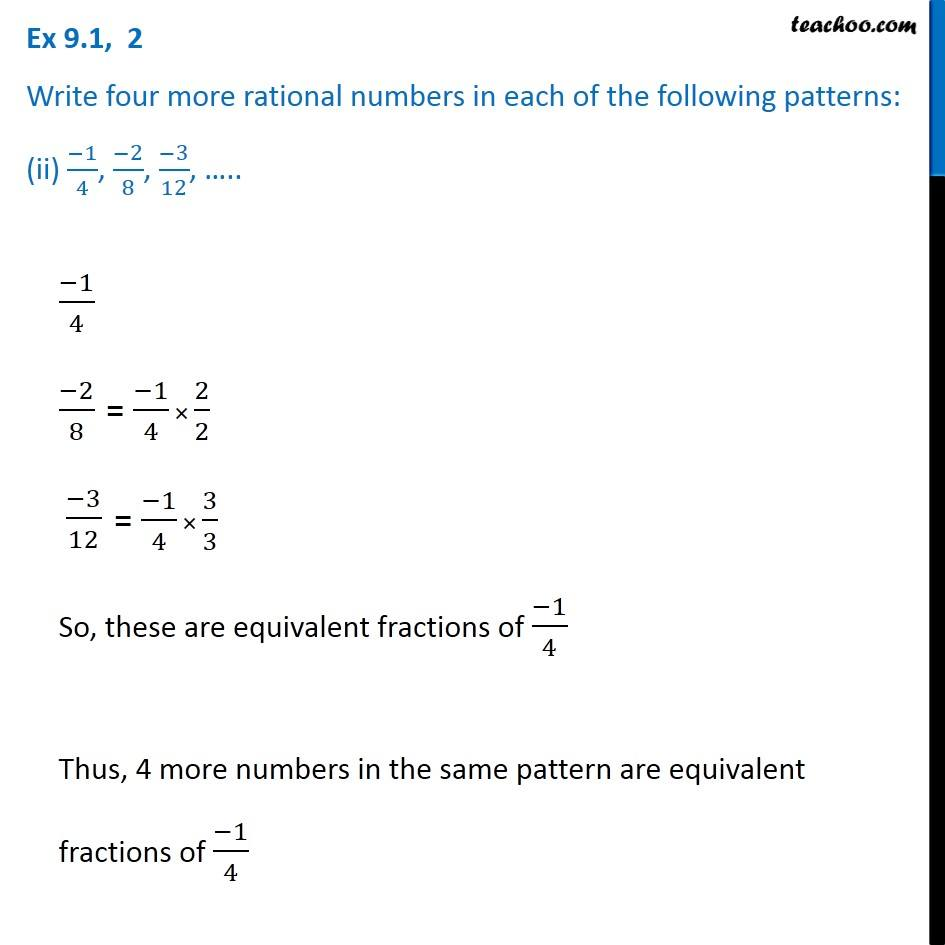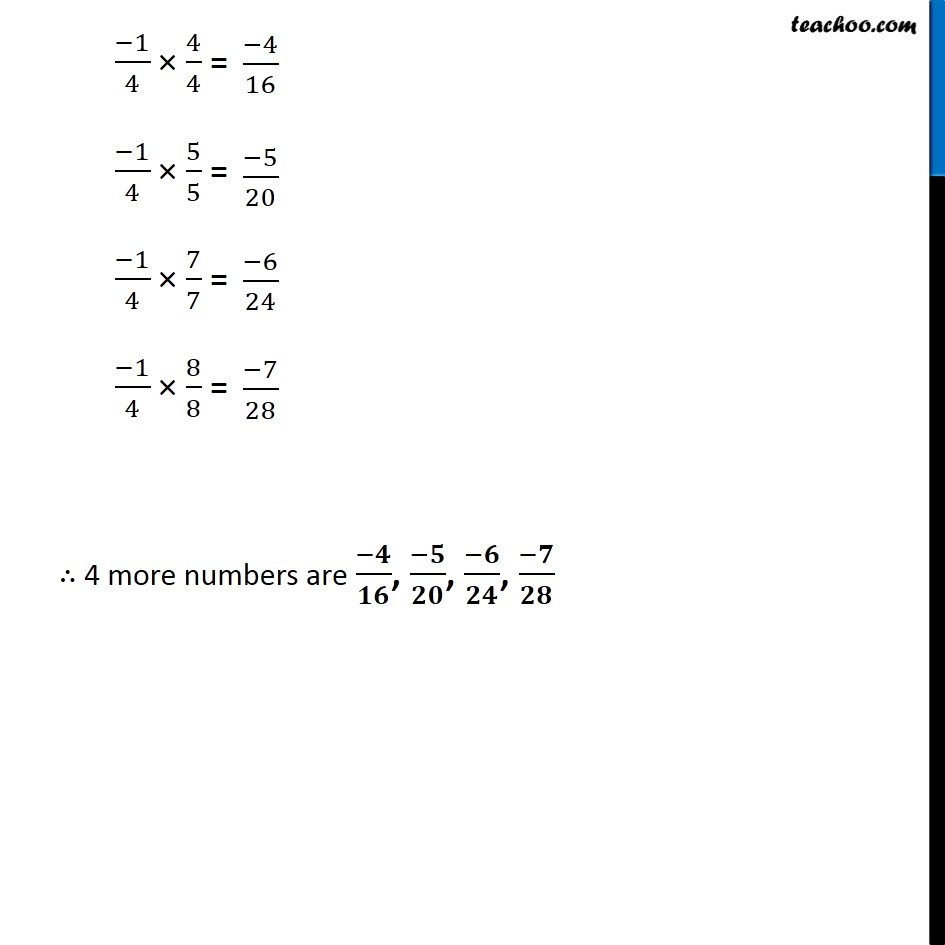Ex 9.1

Chapter 9 Class 7 Rational Numbers
Serial order wiseIntroducing your new favourite teacher - Teachoo Black, at only ₹83 per month

### Transcript

Ex 9.1, 2 Write four more rational numbers in each of the following patterns: (ii) (−1)/4, (−2)/8, (−3)/12, ….. (−1)/4 (−2)/8 (−3)/12 So, these are equivalent fractions of (−1)/4 Thus, 4 more numbers in the same pattern are equivalent fractions of (−1)/4 (−1)/4 × 4/4 = (−1)/4 × 5/5 = (−1)/4 × 7/7 = (−1)/4 × 8/8 = ∴ 4 more numbers are (−𝟒)/𝟏𝟔, (−𝟓)/𝟐𝟎, (−𝟔)/𝟐𝟒, (−𝟕)/𝟐𝟖